Molecular Orbital Theory

Valence Bond Model vs. Molecular Orbital Theory

Because arguments based on atomic orbitals focus on the bonds formed between valence electrons on an atom, they are often said to involve a valence-bond theory.

The valence-bond model can't adequately explain the fact that some molecules contains two equivalent bonds with a bond order between that of a single bond and a double bond. The best it can do is suggest that these molecules are mixtures, or hybrids, of the two Lewis structures that can be written for these molecules.

This problem, and many others, can be overcome by using a more sophisticated model of bonding based on molecular orbitals. Molecular orbital theory is more powerful than valence-bond theory because the orbitals reflect the geometry of the molecule to which they are applied. But this power carries a significant cost in terms of the ease with which the model can be visualized.Forming Molecular Orbitals

Molecular orbitals are obtained by combining the atomic orbitals on the atoms in the molecule. Consider the H2 molecule, for example. One of the molecular orbitals in this molecule is constructed by adding the mathematical functions for the two 1s atomic orbitals that come together to form this molecule. Another orbital is formed by subtracting one of these functions from the other, as shown in the figure below.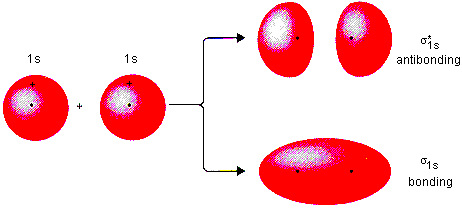One of these orbitals is called a bonding molecular orbital because electrons in this orbital spend most of their time in the region directly between the two nuclei. It is called a sigma () molecular orbital because it looks like an s orbital when viewed along the H-H bond. Electrons placed in the other orbital spend most of their time away from the region between the two nuclei. This orbital is therefore an antibonding, or sigma star (*), molecular orbital.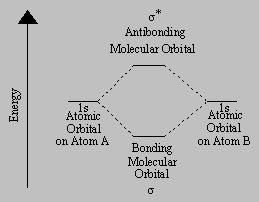Thebonding molecular orbital concentrates electrons in the region directly between the two nuclei. Placing an electron in this orbital therefore stabilizes the H2 molecule. Since the* antibonding molecular orbital forces the electron to spend most of its time away from the area between the nuclei, placing an electron in this orbital makes the molecule less stable.

Electrons are added to molecular orbitals, one at a time, starting with the lowest energy molecular orbital. The two electrons associated with a pair of hydrogen atoms are placed in the lowest energy, orbonding, molecular orbital, as shown in the figure below. This diagram suggests that the energy of an H2 molecule is lower than that of a pair of isolated atoms. As a result, the H2 molecule is more stable than a pair of isolated atoms.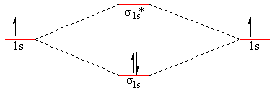Using the Molecular Orbital Model to Explain Why Some Molecules Do Not Exist

This molecular orbital model can be used to explain why He2 molecules don't exist. Combining a pair of helium atoms with 1s2 electron configurations would produce a molecule with a pair of electrons in both thebonding and the* antibonding molecular orbitals. The total energy of an He2 molecule would be essentially the same as the energy of a pair of isolated helium atoms, and there would be nothing to hold the helium atoms together to form a molecule.

The fact that an He2 molecule is neither more nor less stable than a pair of isolated helium atoms illustrates an important principle: The core orbitals on an atom make no contribution to the stability of the molecules that contain this atom. The only orbitals that are important in our discussion of molecular orbitals are those formed when valence-shell orbitals are combined. The molecular orbital diagram for an O2 molecule would therefore ignore the 1s electrons on both oxygen atoms and concentrate on the interactions between the 2s and 2p valence orbitals.Molecular Orbitals of the Second Energy Level

The 2s orbitals on one atom combine with the 2s orbitals on another to form a2s bonding and a2s* antibonding molecular orbital, just like the1s and1s* orbitals formed from the 1s atomic orbitals. If we arbitrarily define the Z axis of the coordinate system for the O2 molecule as the axis along which the bond forms, the 2pz orbitals on the adjacent atoms will meet head-on to form a2p bonding and a2p* antibonding molecular orbital, as shown in the figure below. These are called sigma orbitals because they look like s orbitals when viewed along the oxygen-oxygen bond.The 2px orbitals on one atom interact with the 2px orbitals on the other to form molecular orbitals that have a different shape, as shown in the figure below. These molecular orbitals are called pi () orbitals because they look like p orbitals when viewed along the bond. Whereasand* orbitals concentrate the electrons along the axis on which the nuclei of the atoms lie,and* orbitals concentrate the electrons either above or below this axis.The 2px atomic orbitals combine to form ax bonding molecular orbital and ax* antibonding molecular orbital. The same thing happens when the 2py orbitals interact, only in this case we get ay and ay* antibonding molecular orbital. Because there is no difference between the energies of the 2px and 2py atomic orbitals, there is no difference between the energies of thex andy or thex* andy* molecular orbitals.

The interaction of four valence atomic orbitals on one atom (2s, 2px, 2py and 2pz) with a set of four atomic orbitals on another atom leads to the formation of a total of eight molecular orbitals:2s,2s*,2p,2p*,x,y,x*, andy*.

There is a significant difference between the energies of the 2s and 2p orbitals on an atom. As a result, the2s and*2s orbitals both lie at lower energies than the2p,2p*,x,y,x*, andy* orbitals. To sort out the relative energies of the six molecular orbitals formed when the 2p atomic orbitals on a pair of atoms are combined, we need to understand the relationship between the strength of the interaction between a pair of orbitals and the relative energies of the molecular orbitals they form.

Because they meet head-on, the interaction between the 2pz orbitals is stronger than the interaction between the 2px or 2py orbitals, which meet edge-on. As a result, the2p orbital lies at a lower energy than thex andy orbitals, and the2p* orbital lies at higher energy than thex* andy* orbitals, as shown in the figure below.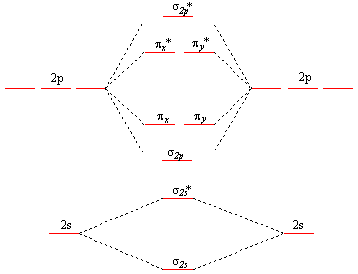Unfortunately an interaction is missing from this model. It is possible for the 2s orbital on one atom to interact with the 2pz orbital on the other. This interaction introduces an element of s-p mixing, or hybridization, into the molecular orbital theory. The result is a slight change in the relative energies of the molecular orbitals, to give the diagram shown in the figure below. Experiments have shown that O2 and F2 are best described by the model in the figure above, but B2, C2, and N2 are best described by a model that includes hybridization, as shown in the figure below.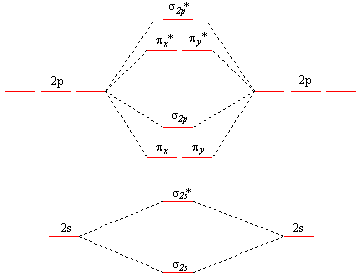Bond Order

The number of bonds between a pair of atoms is called the bond order. Bond orders can be calculated from Lewis structures, which are the heart of the valence-bond model. Oxygen, for example, has a bond order of two.When there is more than one Lewis structure for a molecule, the bond order is an average of these structures. The bond order in sulfur dioxide, for example, is 1.5the average of an S-O single bond in one Lewis structure and an S=O double bond in the other.In molecular orbital theory, we calculate bond orders by assuming that two electrons in a bonding molecular orbital contribute one net bond and that two electrons in an antibonding molecular orbital cancel the effect of one bond. We can calculate the bond order in the O2 molecule by noting that there are eight valence electrons in bonding molecular orbitals and four valence electrons in antibonding molecular orbitals in the electron configuration of this molecule. Thus, the bond order is two.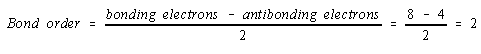Although the Lewis structure and molecular orbital models of oxygen yield the same bond order, there is an important difference between these models. The electrons in the Lewis structure are all paired, but there are two unpaired electrons in the molecular orbital description of the molecule. As a result, we can test the predictions of these theories by studying the effect of a magnetic field on oxygen.

Atoms or molecules in which the electrons are paired are diamagneticrepelled by both poles of a magnetic. Those that have one or more unpaired electrons are paramagneticattracted to a magnetic field. Liquid oxygen is attracted to a magnetic field and can actually bridge the gap between the poles of a horseshoe magnet. The molecular orbital model of O2 is therefore superior to the valence-bond model, which cannot explain this property of oxygen.